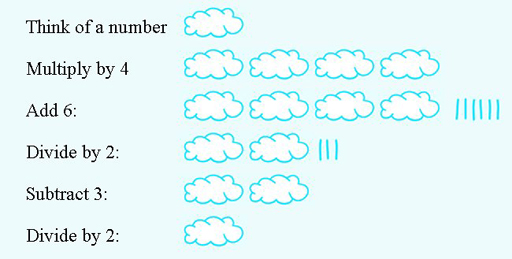Science, Maths & Technology

### Become an OU studentSucceed with maths: part 2

Start this free course now. Just create an account and sign in. Enrol and complete the course for a free statement of participation or digital badge if available.

# 2.2 Another number trick

Here is another number trick which uses the same techniques you learned in the last section to see how it works.

## Activity 6 Another number trick

Timing: Activity 6 Another number trick

Try the following trick several times; make a note of the number you started with and your final answer each time:

• Think of a number between 1 and 10 (this will work with numbers greater than 10, but the restriction is to keep the arithmetic manageable).
• Multiply by 4.
• Divide by 2.
• Subtract 3.
• Divide by 2.

What do you notice about the answer? See if you can explain why this happens, either by using a diagram or by writing down the expressions for the answer at each stage, replacing your initial number with n again.

You should find that this time the answer is always the number you chose at the start. Both ways of showing why the number trick works are shown below.Figure 6 Graphic representation of how the number trick works
Table 2 Table format showing how the number trick works
InstructionExpression
Think of a number between 1 and 10
Multiply by 4